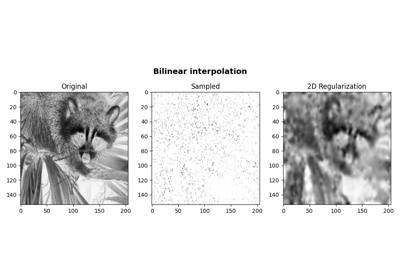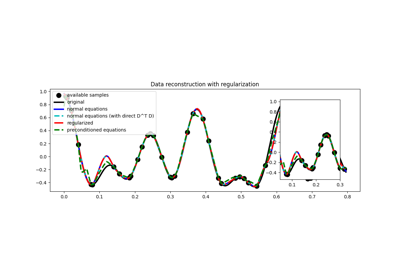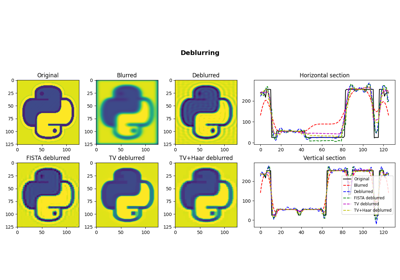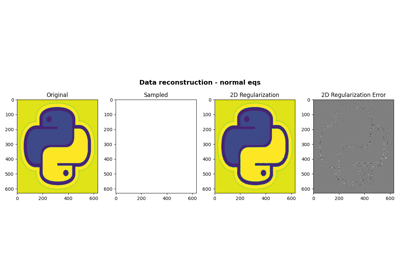# pylops.optimization.leastsquares.NormalEquationsInversion¶

pylops.optimization.leastsquares.NormalEquationsInversion(Op, Regs, data, Weight=None, dataregs=None, epsI=0, epsRs=None, x0=None, returninfo=False, NRegs=None, epsNRs=None, **kwargs_solver)[source]

Inversion of normal equations.

Solve the regularized normal equations for a system of equations given the operator Op, a data weighting operator Weight and optionally a list of regularization terms Regs and/or NRegs.

Parameters: Op : pylops.LinearOperator Operator to invert Regs : list Regularization operators (None to avoid adding regularization) data : numpy.ndarray Data Weight : pylops.LinearOperator, optional Weight operator dataregs : list, optional Regularization data (must have the same number of elements as Regs) epsI : float, optional Tikhonov damping epsRs : list, optional Regularization dampings (must have the same number of elements as Regs) x0 : numpy.ndarray, optional Initial guess returninfo : bool, optional Return info of CG solver NRegs : list Normal regularization operators (None to avoid adding regularization). Such operators must apply the chain of the forward and the adjoint in one go. This can be convenient in cases where a faster implementation is available compared to applying the forward followed by the adjoint. epsNRs : list, optional Regularization dampings for normal operators (must have the same number of elements as NRegs) **kwargs_solver Arbitrary keyword arguments for chosen solver (scipy.sparse.linalg.cg and pylops.optimization.solver.cg are used as default for numpy and cupy data, respectively) Note When user does not supply atol, it is set to “legacy”. xinv : numpy.ndarray Inverted model. istop : int Convergence information: 0: successful exit >0: convergence to tolerance not achieved, number of iterations <0: illegal input or breakdown

RegularizedInversion
Regularized inversion
PreconditionedInversion
Preconditioned inversion

Notes

Solve the following normal equations for a system of regularized equations given the operator $$\mathbf{Op}$$, a data weighting operator $$\mathbf{W}$$, a list of regularization terms ($$\mathbf{R}_i$$ and/or $$\mathbf{N}_i$$), the data $$\mathbf{d}$$ and regularization data $$\mathbf{d}_{\mathbf{R}_i}$$, and the damping factors $$\epsilon_I$$, $$\epsilon_{\mathbf{R}_i}$$ and $$\epsilon_{\mathbf{N}_i}$$:

$( \mathbf{Op}^T \mathbf{W} \mathbf{Op} + \sum_i \epsilon_{\mathbf{R}_i}^2 \mathbf{R}_i^T \mathbf{R}_i + \sum_i \epsilon_{\mathbf{N}_i}^2 \mathbf{N}_i + \epsilon_I^2 \mathbf{I} ) \mathbf{x} = \mathbf{Op}^T \mathbf{W} \mathbf{d} + \sum_i \epsilon_{\mathbf{R}_i}^2 \mathbf{R}_i^T \mathbf{d}_{\mathbf{R}_i}$

Note that the data term of the regularizations $$\mathbf{N}_i$$ is implicitly assumed to be zero.

## Examples using pylops.optimization.leastsquares.NormalEquationsInversion¶Bilinear Interpolation03. Solvers05. Image deblurring06. 2D Interpolation